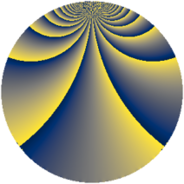# Properties

 Label 729.1.fLevel $729$ Weight $1$ Character orbit 729.f Rep. character $\chi_{729}(80,\cdot)$ Character field $\Q(\zeta_{18})$ Dimension $6$ Newform subspaces $1$ Sturm bound $81$ Trace bound $0$

# Related objects

## Defining parameters

 Level: $$N$$ $$=$$ $$729 = 3^{6}$$ Weight: $$k$$ $$=$$ $$1$$ Character orbit: $$[\chi]$$ $$=$$ 729.f (of order $$18$$ and degree $$6$$) Character conductor: $$\operatorname{cond}(\chi)$$ $$=$$ $$27$$ Character field: $$\Q(\zeta_{18})$$ Newform subspaces: $$1$$ Sturm bound: $$81$$ Trace bound: $$0$$

## Dimensions

The following table gives the dimensions of various subspaces of $$M_{1}(729, [\chi])$$.

Total New Old
Modular forms 114 24 90
Cusp forms 6 6 0
Eisenstein series 108 18 90

The following table gives the dimensions of subspaces with specified projective image type.

$$D_n$$ $$A_4$$ $$S_4$$ $$A_5$$
Dimension 6 0 0 0

## Trace form

 $$6 q + O(q^{10})$$ $$6 q + 3 q^{19} - 6 q^{28} + 3 q^{37} - 3 q^{64} - 6 q^{73} - 3 q^{91} + O(q^{100})$$

## Decomposition of $$S_{1}^{\mathrm{new}}(729, [\chi])$$ into newform subspaces

Label Dim $A$ Field Image CM RM Traces $q$-expansion
$a_{2}$ $a_{3}$ $a_{5}$ $a_{7}$
729.1.f.a $6$ $0.364$ $$\Q(\zeta_{18})$$ $D_{3}$ $$\Q(\sqrt{-3})$$ None $$0$$ $$0$$ $$0$$ $$0$$ $$q-\zeta_{18}q^{4}-\zeta_{18}^{8}q^{7}-\zeta_{18}^{4}q^{13}+\cdots$$